Introduction

The teacher greets the learners and ask them to sit at their desks.

Based on their prior knowledge, the teacher ask the following questions.

1. The teacher asks the learners to empty their desks (put books away).

2. The teachers ask what shape their desks are and how they know it is that specific shape.

This exercise leads to an introduction for the proof of properties of quadrilaterals

(Learners will think critically as to why their desks are square-shaped)

Learner response

1.Learners put their books away. Their desks are now empty

2. Rectangle, square, etc. and reason with the teacher why they think their desks are that specific shape, i.e., square

Instruction

1. Do the following in your workbooks:

1.      DELM is a parallelogram with DM = 50 and = 150°. Determine the length of EL and the sizes of , and .  (10)

2.      DELM is a parallelogram. Solve for 𝑥 and determine the co-interior angles.                                                          (10)

3.      In trapezium ABCD, 𝐴̂=𝐷̂ = 70° en EC = DC. Prove that ABCE is a parallelogram                                                 (15)

Total 

Process

The teacher goes through the properties of quadrilaterals as the learners will need it to do the task

A quadrilateral is a closed shape consisting of four straight line segments.

A parallelogram is a quadrilateral with both pairs of opposite sides parallel.

§  Both pairs of opposite sides are equal in length.

§  Both pairs of opposite angles are equal.

§  Both diagonals bisect each other.

A rectangle is a parallelogram that has all four angles equal to 90°

§  Both pairs of opposite sides are parallel.

§  Both pairs of opposite sides are equal in length.

§  The diagonals bisect each other.

§  The diagonals are equal in length.

§  All interior angles are equal to 90°

A rhombus is a parallelogram that has all four sides equal in length.

§  Both pairs of opposite sides are parallel.

§  All sides are equal in length.

§  Both pairs of opposite angles are equal.

§  The diagonals bisect each other at 90°

§  The diagonals of a rhombus bisect both pairs of opposite angles.

• A square is a rhombus that has all four interior angles equal to 90°

§  Both pairs of opposite sides are parallel.

§  The diagonals bisect each other at 90°

§  All interior angles are equal to 90° § The diagonals are equal in length.

§  The diagonals bisect both pairs of interior opposite angles (i.e. all are 45°)

A trapezium is a quadrilateral with one pair of opposite sides parallel.

A kite is a quadrilateral with two pairs of adjacent sides equal.

§  One pair of opposite angles are equal (the angles are between unequal sides).

§  The diagonal between equal sides bisects the other diagonal. § The diagonal between equal sides bisects the interior angles. § The diagonals intersect at 90°.

Evaluation

Learner's task will be marked according to the following marking criteria.

Learners need to share their work and submit via the following email address:

1. Solution

EL = 50     opp. sides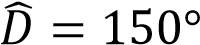Opp. angles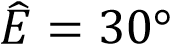Co-interior angles; DE||ML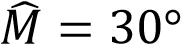Opp. angles

2. Solution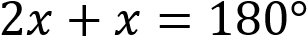Co-interior angles; DM||EL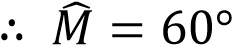Opp. angles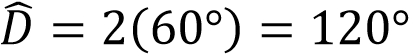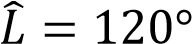Opp. angles

3. Solutionangles opposite equal sides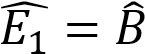Therefore, ABCE is a parallelogram. Both pairs of opposite angles are equal.                 Marks 

Conclusion

After completion of examples, the teacher revise the properties and gives homework.

The teacher greets the learners.

Credits
 1. Basson, M., Botha, C. & Phillips, M. D. 2011. Mind Action Series Mathematics 10 Textbook. Cape Town: Allcopy Publishers.

2. Jenkin, A., Kannemeyer, L., Scheffers, C. & Van Zyl, M. 2012. Siyavula Grade 10 Mathematics.

Teacher Page